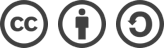## 算法：求最大子序和 C#版本

2019-10-18

### 算法：求最大子序和 C#版本

#### 描述

``````输入: [-2,1,-3,4,-1,2,1,-5,4],

#### 思路

1. 设初始值 最大和ans=第一个元素，初始和为0 ，比较两值，找到最大值。
2. 循环所有元素 ，当sum<=0时，把当前元素的值给sum,同时比较sum和ans的哪个值大。

sum<0时，声明的sum=0,会变更为第一个小于0的值，所以第一次是两个第一个值进行比较，取其中一个就行。

1. 如果sum>0,则计算 sum=sum+当前元素值

#### 算法实现

``````private class Solution
{
public int maxSubArray(int[] nums)
{
int ans = nums;
int sum = 0;
foreach (var num in nums)
{
if (sum > 0)
{
sum += num;
}
else
{
sum = num;
}
ans = Math.Max(ans, sum);
}
return ans;
}
}``````

#### 调用测试

``````private static void Main(string[] args)
{
//int[] nums = new[] {-2, 1, -3, 4, -1, 2, 1, -5, 4};
int[] nums = new[] {-2, -3,-1,  -5};

int result = new Solution().maxSubArray(nums);

Console.WriteLine(result);